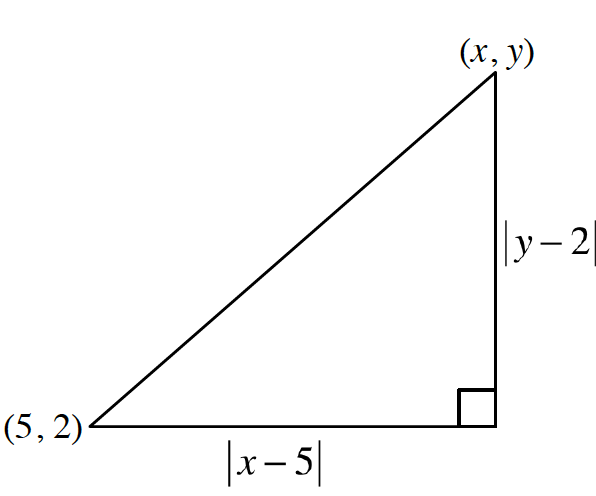### Home > PC3 > Chapter 10 > Lesson 10.1.1 > Problem10-6

10-6.

Determine the distance between each of the following pairs of points.

1. $(−6, 9)$ and $(2, −4)$

Plot the points and use them to create a right triangle where the segment connecting the points is the hypotenuse. Determine the lengths of the legs, then use the Pythagorean Theorem to calculate the distance between the points.

1. $(x, y)$ and $(5, 2)$